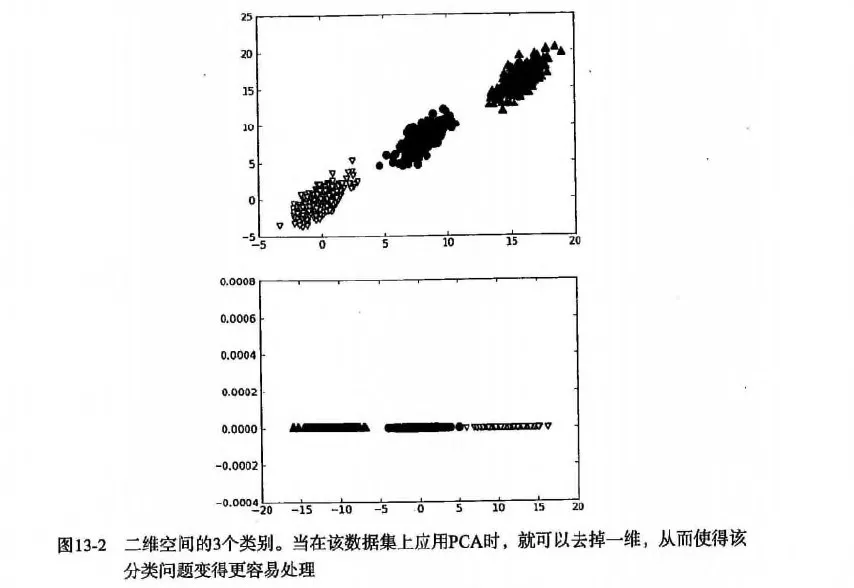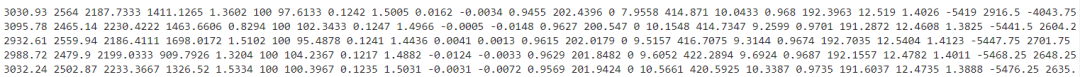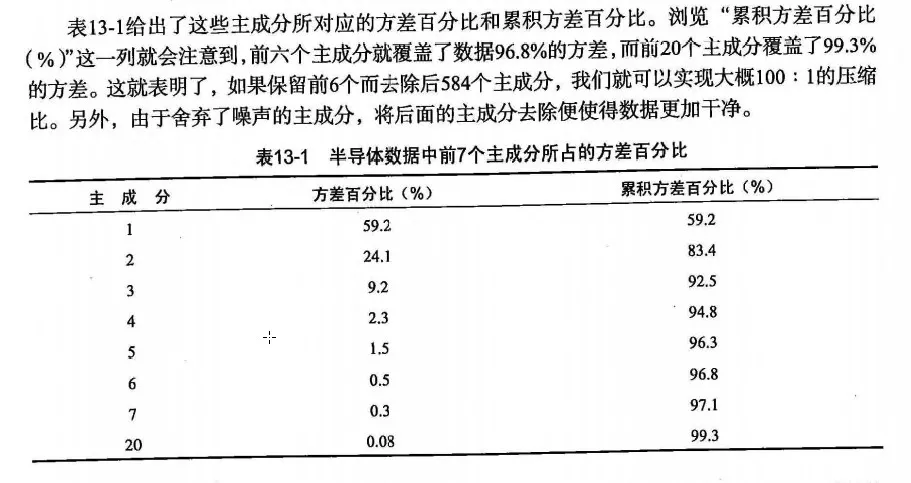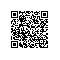# 怎样用PCA技术简化数据，你造吗？

### 1、降维技术

#### 四 PCA降维技术

1、主成分分析(Principal Component Analysis, PCA）

2、因子分析(Factor Analysis)

那么隐变量的数据可能比观察数据的数目少，也就说通过找到隐变量就可以实现数据的降维。


3、独立成分分析(Independ Component Analysis, ICA)

ICA 是假设数据是从 N 个数据源混合组成的，这一点和因子分析有些类似，这些数据源之间在统计上是相互独立的，而在 PCA 中只假设数据是不 相关（线性关系）的。

### 02、PCA

#### 三 PCA原理

1、PCA 工作原理

2、为什么正交？3、PCA 优缺点

### 3、项目案例：对半导体数据进行降维处理

#### 二 开发流程

1、收集数据：提供文本文件2、准备数据：将value为NaN的求均值

def replaceNanWithMean():
numFeat = shape(datMat)
for i in range(numFeat):
# 对value不为NaN的求均值
# .A 返回矩阵基于的数组
meanVal = mean(datMat[nonzero(~isnan(datMat[:, i].A)), i])
# 将value为NaN的值赋值为均值
datMat[nonzero(isnan(datMat[:, i].A)),i] = meanVal
return datMat

3、分析数据：统计分析 N 的阈值4、PCA 数据降维

def pca(dataMat, topNfeat=9999999):
"""pca

Args:
dataMat   原数据集矩阵
topNfeat  应用的N个特征
Returns:
lowDDataMat  降维后数据集
reconMat     新的数据集空间
"""

# 计算每一列的均值
meanVals = mean(dataMat, axis=0)
# print 'meanVals', meanVals

# 每个向量同时都减去 均值
meanRemoved = dataMat - meanVals
# print 'meanRemoved=', meanRemoved

# cov协方差=[(x1-x均值)*(y1-y均值)+(x2-x均值)*(y2-y均值)+...+(xn-x均值)*(yn-y均值)+]/(n-1)
'''
方差：（一维）度量两个随机变量关系的统计量
协方差： （二维）度量各个维度偏离其均值的程度
协方差矩阵：（多维）度量各个维度偏离其均值的程度

当 cov(X, Y)>0时，表明X与Y正相关；(X越大，Y也越大；X越小Y，也越小。这种情况，我们称为“正相关”。)
当 cov(X, Y)<0时，表明X与Y负相关；
当 cov(X, Y)=0时，表明X与Y不相关。
'''
covMat = cov(meanRemoved, rowvar=0)

# eigVals为特征值， eigVects为特征向量
eigVals, eigVects = linalg.eig(mat(covMat))
# print 'eigVals=', eigVals
# print 'eigVects=', eigVects
# 对特征值，进行从小到大的排序，返回从小到大的index序号
# 特征值的逆序就可以得到topNfeat个最大的特征向量
'''
>>> x = np.array([3, 1, 2])
>>> np.argsort(x)
array([1, 2, 0])  # index,1 = 1; index,2 = 2; index,0 = 3
>>> y = np.argsort(x)
>>> y[::-1]
array([0, 2, 1])
>>> y[:-3:-1]
array([0, 2])  # 取出 -1, -2
>>> y[:-6:-1]
array([0, 2, 1])
'''
eigValInd = argsort(eigVals)
# print 'eigValInd1=', eigValInd

# -1表示倒序，返回topN的特征值[-1 到 -(topNfeat+1) 但是不包括-(topNfeat+1)本身的倒叙]
eigValInd = eigValInd[:-(topNfeat+1):-1]
# print 'eigValInd2=', eigValInd
# 重组 eigVects 最大到最小
redEigVects = eigVects[:, eigValInd]
# print 'redEigVects=', redEigVects.T
# 将数据转换到新空间
# --- (1567, 590) (590, 20)
# print "---", shape(meanRemoved), shape(redEigVects)
lowDDataMat = meanRemoved * redEigVects
reconMat = (lowDDataMat * redEigVects.T) + meanVals
# print 'lowDDataMat=', lowDDataMat
# print 'reconMat=', reconMat
return lowDDataMat, reconMat
##项目完整代码：

#!/usr/bin/python
# coding:utf8

'''
Created on Jun 1, 2011
Update  on 2017-12-20
@author: Peter Harrington/片刻
《机器学习实战》更新地址：https://github.com/apachecn/MachineLearning
'''
from numpy import *
import matplotlib.pyplot as plt
print(__doc__)

fr = open(fileName)
stringArr = [line.strip().split(delim) for line in fr.readlines()]
datArr = [list(map(float, line)) for line in stringArr]
#注意这里和python2的区别，需要在map函数外加一个list（），否则显示结果为 map at 0x3fed1d0
return mat(datArr)

def pca(dataMat, topNfeat=9999999):
"""pca

Args:
dataMat   原数据集矩阵
topNfeat  应用的N个特征
Returns:
lowDDataMat  降维后数据集
reconMat     新的数据集空间
"""

# 计算每一列的均值
meanVals = mean(dataMat, axis=0)
# print('meanVals', meanVals)

# 每个向量同时都减去 均值
meanRemoved = dataMat - meanVals
# print('meanRemoved=', meanRemoved)

# cov协方差=[(x1-x均值)*(y1-y均值)+(x2-x均值)*(y2-y均值)+...+(xn-x均值)*(yn-y均值)+]/(n-1)
'''
方差：（一维）度量两个随机变量关系的统计量
协方差： （二维）度量各个维度偏离其均值的程度
协方差矩阵：（多维）度量各个维度偏离其均值的程度

当 cov(X, Y)>0时，表明X与Y正相关；(X越大，Y也越大；X越小Y，也越小。这种情况，我们称为“正相关”。)
当 cov(X, Y)<0时，表明X与Y负相关；
当 cov(X, Y)=0时，表明X与Y不相关。
'''
covMat = cov(meanRemoved, rowvar=0)

# eigVals为特征值， eigVects为特征向量
eigVals, eigVects = linalg.eig(mat(covMat))
# print('eigVals=', eigVals)
# print('eigVects=', eigVects)
# 对特征值，进行从小到大的排序，返回从小到大的index序号
# 特征值的逆序就可以得到topNfeat个最大的特征向量
'''
>>> x = np.array([3, 1, 2])
>>> np.argsort(x)
array([1, 2, 0])  # index,1 = 1; index,2 = 2; index,0 = 3
>>> y = np.argsort(x)
>>> y[::-1]
array([0, 2, 1])
>>> y[:-3:-1]
array([0, 2])  # 取出 -1, -2
>>> y[:-6:-1]
array([0, 2, 1])
'''
eigValInd = argsort(eigVals)
# print('eigValInd1=', eigValInd)

# -1表示倒序，返回topN的特征值[-1 到 -(topNfeat+1) 但是不包括-(topNfeat+1)本身的倒叙]
eigValInd = eigValInd[:-(topNfeat+1):-1]
# print('eigValInd2=', eigValInd)
# 重组 eigVects 最大到最小
redEigVects = eigVects[:, eigValInd]
# print('redEigVects=', redEigVects.T)
# 将数据转换到新空间
# print( "---", shape(meanRemoved), shape(redEigVects))
lowDDataMat = meanRemoved * redEigVects
reconMat = (lowDDataMat * redEigVects.T) + meanVals
# print('lowDDataMat=', lowDDataMat)
# print('reconMat=', reconMat)
return lowDDataMat, reconMat

def replaceNanWithMean():
numFeat = shape(datMat)
for i in range(numFeat):
# 对value不为NaN的求均值
# .A 返回矩阵基于的数组
meanVal = mean(datMat[nonzero(~isnan(datMat[:, i].A)), i])
# 将value为NaN的值赋值为均值
datMat[nonzero(isnan(datMat[:, i].A)),i] = meanVal
return datMat

def show_picture(dataMat, reconMat):
fig = plt.figure()
ax.scatter(dataMat[:, 0].flatten().A, dataMat[:, 1].flatten().A, marker='^', s=90)
ax.scatter(reconMat[:, 0].flatten().A, reconMat[:, 1].flatten().A, marker='o', s=50, c='red')
plt.show()

def analyse_data(dataMat):
meanVals = mean(dataMat, axis=0)
meanRemoved = dataMat-meanVals
covMat = cov(meanRemoved, rowvar=0)
eigvals, eigVects = linalg.eig(mat(covMat))
eigValInd = argsort(eigvals)

topNfeat = 20
eigValInd = eigValInd[:-(topNfeat+1):-1]
cov_all_score = float(sum(eigvals))
sum_cov_score = 0
for i in range(0, len(eigValInd)):
line_cov_score = float(eigvals[eigValInd[i]])
sum_cov_score += line_cov_score
'''
我们发现其中有超过20%的特征值都是0。
这就意味着这些特征都是其他特征的副本，也就是说，它们可以通过其他特征来表示，而本身并没有提供额外的信息。

最前面15个值的数量级大于10^5，实际上那以后的值都变得非常小。
这就相当于告诉我们只有部分重要特征，重要特征的数目也很快就会下降。

最后，我们可能会注意到有一些小的负值，他们主要源自数值误差应该四舍五入成0.
'''
print('主成分：%s, 方差占比：%s%%, 累积方差占比：%s%%' % (format(i+1, '2.0f'), format(line_cov_score/cov_all_score*100, '4.2f'), format(sum_cov_score/cov_all_score*100, '4.1f')))

if __name__ == "__main__":
# # 加载数据，并转化数据类型为float
# # 只需要1个特征向量
# lowDmat, reconMat = pca(dataMat, 1)
# # 只需要2个特征向量，和原始数据一致，没任何变化
# # lowDmat, reconMat = pca(dataMat, 2)
# # print(shape(lowDmat))
# show_picture(dataMat, reconMat)

# 利用PCA对半导体制造数据降维
dataMat = replaceNanWithMean()
print(shape(dataMat))
# 分析数据
analyse_data(dataMat)
# lowDmat, reconMat = pca(dataMat, 20)
# print(shape(lowDmat))
# show_picture(dataMat, reconMat)

### 4、要点补充使用钉钉扫一扫加入圈子
+ 订阅